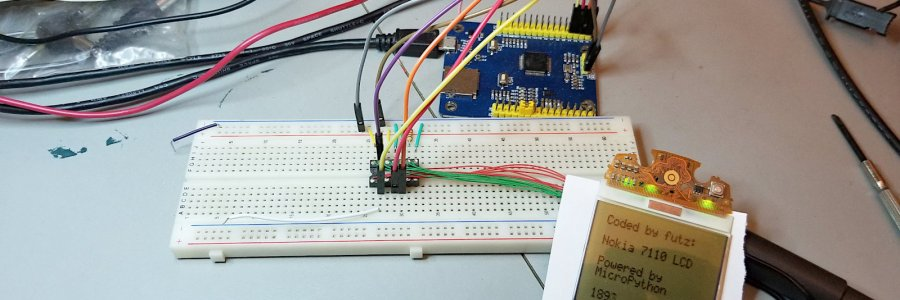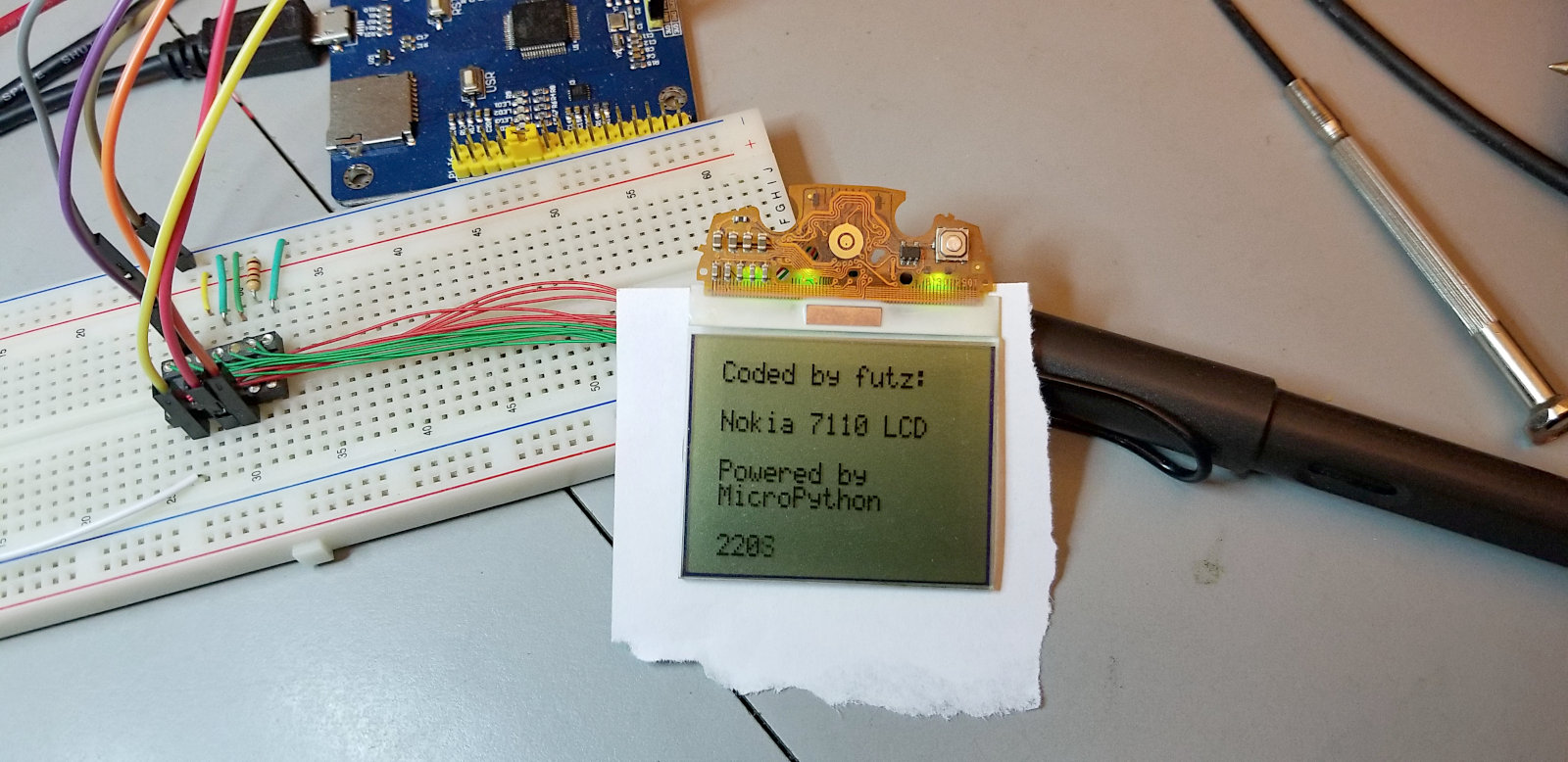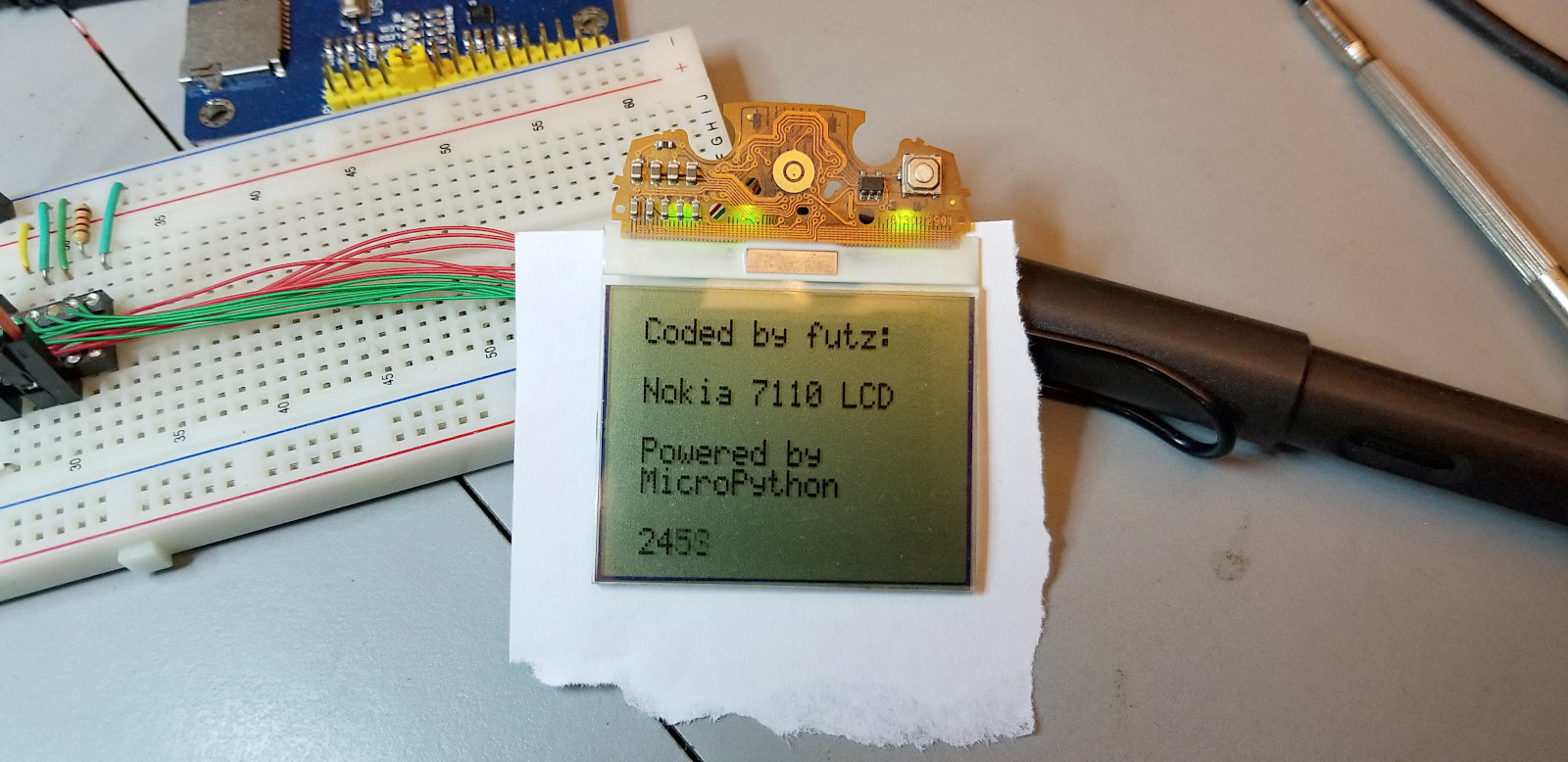#### Pyboard driving Nokia 7110 LCDI ported my 7110 LCD C code for PIC to MicroPython on the PyBoard. It wasn't very difficult. Yet again, most of the trouble I had was due to having to learn Python as I ported the code. Not a big deal - Python isn't difficult to learn.

I wasn't sure about text formatting for my font array in Python, so I turned it into the big blob (one long line) you see below. Now that I have the code working I may tinker a bit and see if I can get it formatted to be more readable.Video of the 7110 LCD driven by the PyBoard:I fought with my graphic code for quite a while before finally figuring out the Python way. I could not get it to work properly for the longest time. First problem was figuring out that the way Python handles global variables is... odd. Nothin wrong with it - it's just unusual.

Then I had to figure out how to deal with negative numbers, which Python does completely differently than C does. I eventually realized that I was only using the negative numbers to do a ones complement for a mask to clear pixels, so I didn't need to do it the C way at all. Rewrote those bits of code and cured that problem. Easy, but took forever to find the problem.

Then I decided to change from using arrays to native Python lists for the frame buffers. Easier, and works just as well.

The code works well. It's not blazing fast, but good enough for what it is. If I cared there are things I could do to improve the speed some.

Here's video of the demo:

Here's the MicroPython PyBoard code to do text on the 7110 LCD. Scroll down past it to see the graphic demo code.:

``````from pyb import Pin
import array as arr

CS = Pin('X4',Pin.OUT_PP)
DC = Pin('X3',Pin.OUT_PP)
SCLK = Pin('X2',Pin.OUT_PP)
SDATA = Pin('X1',Pin.OUT_PP)

#ascii font - Space 32 (\$20) thru DEL 127 (\$7f)
Alpha1 = arr.array('B',[0x00,0x00,0x00,0x00,0x00,0x00,0x00,0x5f,0x00,0x00,0x00,0x07,0x00,0x07,0x00,0x14,0x7f,0x14,0x7f,0x14,0x24,0x2a,0x7f,0x2a,0x12,0x23,0x13,0x08,0x64,0x62,0x36,0x49,0x55,0x22,0x50,0x00,0x05,0x03,0x00,0x00,0x00,0x1c,0x22,0x41,0x00,0x00,0x41,0x22,0x1c,0x00,0x14,0x08,0x3e,0x08,0x14,0x08,0x08,0x3e,0x08,0x08,0x00,0x50,0x30,0x00,0x00,0x08,0x08,0x08,0x08,0x08,0x00,0x60,0x60,0x00,0x00,0x20,0x10,0x08,0x04,0x02,0x3e,0x51,0x49,0x45,0x3e,0x00,0x42,0x7f,0x40,0x00,0x42,0x61,0x51,0x49,0x46,0x21,0x41,0x45,0x4b,0x31,0x18,0x14,0x12,0x7f,0x10,0x27,0x45,0x45,0x45,0x39,0x3c,0x4a,0x49,0x49,0x30,0x01,0x71,0x09,0x05,0x03,0x36,0x49,0x49,0x49,0x36,0x06,0x49,0x49,0x29,0x1e,0x00,0x36,0x36,0x00,0x00,0x00,0x56,0x36,0x00,0x00,0x08,0x14,0x22,0x41,0x00,0x14,0x14,0x14,0x14,0x14,0x00,0x41,0x22,0x14,0x08,0x02,0x01,0x51,0x09,0x06,0x32,0x49,0x79,0x41,0x3e,0x7e,0x11,0x11,0x11,0x7e,0x7f,0x49,0x49,0x49,0x36,0x3e,0x41,0x41,0x41,0x22,0x7f,0x41,0x41,0x22,0x1c,0x7f,0x49,0x49,0x49,0x41,0x7f,0x09,0x09,0x09,0x01,0x3e,0x41,0x49,0x49,0x7a,0x7f,0x08,0x08,0x08,0x7f,0x00,0x41,0x7f,0x41,0x00,0x20,0x40,0x41,0x3f,0x01,0x7f,0x08,0x14,0x22,0x41,0x7f,0x40,0x40,0x40,0x40,0x7f,0x02,0x0c,0x02,0x7f,0x7f,0x04,0x08,0x10,0x7f,0x3e,0x41,0x41,0x41,0x3e,0x7f,0x09,0x09,0x09,0x06,0x3e,0x41,0x51,0x21,0x5e,0x7f,0x09,0x19,0x29,0x46,0x46,0x49,0x49,0x49,0x31,0x01,0x01,0x7f,0x01,0x01,0x3f,0x40,0x40,0x40,0x3f,0x1f,0x20,0x40,0x20,0x1f,0x3f,0x40,0x38,0x40,0x3f,0x63,0x14,0x08,0x14,0x63,0x07,0x08,0x70,0x08,0x07,0x61,0x51,0x49,0x45,0x43,0x00,0x7f,0x41,0x41,0x00,0x02,0x04,0x08,0x10,0x20,0x00,0x41,0x41,0x7f,0x00,0x04,0x02,0x01,0x02,0x04,0x40,0x40,0x40,0x40,0x40,0x00,0x01,0x02,0x04,0x00,0x20,0x54,0x54,0x54,0x78,0x7f,0x48,0x44,0x44,0x38,0x38,0x44,0x44,0x44,0x20,0x38,0x44,0x44,0x48,0x7f,0x38,0x54,0x54,0x54,0x18,0x08,0x7e,0x09,0x01,0x02,0x0c,0x52,0x52,0x52,0x3e,0x7f,0x08,0x04,0x04,0x78,0x00,0x44,0x7d,0x40,0x00,0x20,0x40,0x44,0x3d,0x00,0x7f,0x10,0x28,0x44,0x00,0x00,0x41,0x7f,0x40,0x00,0x7c,0x04,0x18,0x04,0x78,0x7c,0x08,0x04,0x04,0x78,0x38,0x44,0x44,0x44,0x38,0x7c,0x14,0x14,0x14,0x08,0x08,0x14,0x14,0x18,0x7c,0x7c,0x08,0x04,0x04,0x08,0x48,0x54,0x54,0x54,0x20,0x04,0x3f,0x44,0x40,0x20,0x3c,0x40,0x40,0x20,0x7c,0x1c,0x20,0x40,0x20,0x1c,0x3c,0x40,0x30,0x40,0x3c,0x44,0x28,0x10,0x28,0x44,0x0c,0x50,0x50,0x50,0x3c,0x44,0x64,0x54,0x4c,0x44,0x00,0x08,0x36,0x41,0x00,0x00,0x00,0x7f,0x00,0x00,0x00,0x41,0x36,0x08,0x00,0x10,0x08,0x08,0x10,0x08,0x78,0x46,0x41,0x46,0x78])

#outputs a string (up to 16 chars) to any of 8 rows (0 - 7)
def lcd_string(str,row):
page = 0xb0
page = page + row
lcd_send(0x02,0)
for x in range(len(str)):
letter = ord(str[x]) - 0x20
for col in range(5):
lcd_send(Alpha1[(letter*5)+col],1)
lcd_send(0,1)

#outputs a single byte to the display
def lcd_send(cmd,type):
if type:
DC.high()
else:
DC.low()
CS.low()
for x in range(8):
SCLK.low()
SDATA.low()
if cmd & 0x80:
SDATA.high()
SCLK.high()
cmd = cmd << 1
CS.high()

#clear screen
def lcd_cls():
line = 0xb0                 #page address variable
for x in range(9):
lcd_send(0x02,0)
for i in range(0x60):   #write zeros to display RAM
lcd_send(0x00,1)
line += 1

#clear single row
def lcd_row_cls(row):
line = 0xb0 + row
lcd_send(line,0)
lcd_send(0x11,0)
lcd_send(0x02,0)
for x in range(0x60):
lcd_send(0x00,1)

#initialize LCD
def lcd_init():
DC.high()
pyb.delay(2)
CS.high()
pyb.delay(2)
lcd_send(0xa6,0)        #Display: Normal
lcd_send(0xa3,0)        #LCD Bias Settings: 1/7
#lcd_send(0xc0,0)       #Common Output: Upside Down
lcd_send(0x22,0)        #Set the V5 output voltage
lcd_send(0x81,0)        #set Electronic Volume - brightness
lcd_send(0x2f,0)
lcd_send(0x2e,0)        #Power Controller Set: Booster circuit: ON
#Voltage regulator circuit: ON
#Voltage follower circuit: off
lcd_send(0x2f,0)        #Power Controller Set: Voltage follower circuit: ON
#lcd_send(0xe3,0)       #Non-Operation Command
#lcd_send(0x40,0)       #Set the start line
lcd_send(0xaf,0)        #LCD on
lcd_send(0xa4,0)        #Display all points: Normal
lcd_cls()

#------------------------------------------------------
# Begin here
#------------------------------------------------------

lcd_init()
lcd_string("Coded by futz:  ",0)
lcd_string("Nokia 7110 LCD  ",2)
lcd_string("MicroPython     ",5)

for x in range(999999):
lcd_string(str(x),7)
pyb.delay(50)``````

Here is the MicroPython Pyboard code for the simple bouncy line graphic demo above:

``````from pyb import Pin

CS = Pin('X4',Pin.OUT_PP)
DC = Pin('X3',Pin.OUT_PP)
SCLK = Pin('X2',Pin.OUT_PP)
SDATA = Pin('X1',Pin.OUT_PP)

framebuff1 =  * 192
framebuff2 =  * 192
framebuff3 =  * 192
framebuff4 =  * 192
framebuff5 =  * 96

def update():
global framebuff1
global framebuff2
global framebuff3
global framebuff4
global framebuff5
count = 0
page = 0xb0
for x in range(2):
lcd_send(page,0)
lcd_send(0x11,0)
lcd_send(0x02,0)
for i in range(0x60):
outbyte = framebuff1[count]
count += 1
lcd_send(outbyte,1)
page += 1
count = 0
for x in range(2):
lcd_send(page,0)
lcd_send(0x11,0)
lcd_send(0x02,0)
for i in range(0x60):
outbyte = framebuff2[count]
count += 1
lcd_send(outbyte,1)
page += 1
count = 0
for x in range(2):
lcd_send(page,0)
lcd_send(0x11,0)
lcd_send(0x02,0)
for i in range(0x60):
outbyte = framebuff3[count]
count += 1
lcd_send(outbyte,1)
page += 1
count = 0
for x in range(2):
lcd_send(page,0)
lcd_send(0x11,0)
lcd_send(0x02,0)
for i in range(0x60):
outbyte = framebuff4[count]
count += 1
lcd_send(outbyte,1)
page += 1
count = 0
lcd_send(page,0)
lcd_send(0x11,0)
lcd_send(0x02,0)
for i in range(0x60):
outbyte = framebuff1[count]
count += 1
lcd_send(outbyte,1)

def pixel(x,y,color):
global framebuff1
global framebuff2
global framebuff3
global framebuff4
global framebuff5
div = y >> 3
if div < 2:
offset = div * 96 + x
mbit = y - (div << 3)
temp = 1 << mbit
if color == 0:
else:
framebuff1[offset] = y
elif div < 4:
offset = ((div * 96) - 192) + x
mbit = y - (div << 3)
temp = 1 << mbit
if color == 0:
else:
framebuff2[offset] = y
elif div < 6:
offset = ((div * 96)-384) + x
mbit = y - (div << 3)
temp = 1 << mbit
if color == 0:
else:
framebuff3[offset] = y
elif div < 8:
offset = ((div * 96) - 576) + x
mbit = y - (div << 3)
temp = 1 << mbit
if color == 0:
else:
framebuff4[offset] = y
elif div < 9:
offset = ((div * 96) - 768) + x
mbit = y - (div << 3)
temp = 1 << mbit
if color == 0:
else:
framebuff5[offset] = y

def line(x1,y1,x2,y2,color):
steep = abs(y2-y1) > abs(x2-x1)
if(steep):
temp = x1       #swap x1, y1
x1 = y1
y1 = temp
temp = x2       #swap x2, y2
x2 = y2
y2 = temp
deltax = abs(x2 - x1)
deltay = abs(y2 - y1)
error = 0
deltaerror = deltay
x = x1
y = y1
if x1 < x2:
xstep = 1
else:
xstep = -1
if y1 < y2:
ystep = 1
else:
ystep = -1
if steep:
pixel(y,x,color)
else:
pixel(x,y,color)
while x != x2:
x += xstep
error += deltaerror
if (error << 1) > deltax:
y += ystep
error = error - deltax
if steep:
pixel(y,x,color)
else:
pixel(x,y,color)

#outputs a single byte to the display
#type 1 = ascii character
#type 0 = command
def lcd_send(cmd,type):
if type:
DC.high()
else:
DC.low()
CS.low()
for x in range(8):
SCLK.low()
SDATA.low()
if cmd & 0x80:
SDATA.high()
SCLK.high()
cmd = cmd << 1
CS.high()

#clear screen
def lcd_cls():
line = 0xb0                 #page address variable
for x in range(8):
lcd_send(0x02,0)
for i in range(0x60):   #write zeros to display RAM
lcd_send(0x00,1)
line += 1

#initialize LCD
def lcd_init():
DC.high()
pyb.delay(2)
CS.high()
pyb.delay(2)
lcd_send(0xa6,0)        #Display: Normal
lcd_send(0xa3,0)        #LCD Bias Settings: 1/7
#lcd_send(0xc0,0)       #Common Output: Upside Down
lcd_send(0x22,0)        #Set the V5 output voltage
lcd_send(0x81,0)        #set Electronic Volume - brightness
lcd_send(0x2f,0)
lcd_send(0x2e,0)        #Power Controller Set: Booster circuit: ON
#Voltage regulator circuit: ON
#Voltage follower circuit: off
lcd_send(0x2f,0)        #Power Controller Set: Voltage follower circuit: ON
#lcd_send(0xe3,0)       #Non-Operation Command
#lcd_send(0x40,0)       #Set the start line
lcd_send(0xaf,0)        #LCD on
lcd_send(0xa4,0)        #Display all points: Normal
lcd_cls()

#------------------------------------------------------
# Begin here
#------------------------------------------------------

lcd_init()
x = [3,45,90,10]
y = [2,50,30,10]
px0 = x; py0 = y; px1 = x; py1 = y
px2 = x; py2 = y; px3 = x; py3 = y
dx0 = 3; dy0 = -2; dx1 = -3; dy1 = 3; dx2 = -3; dy2 = 3; dx3 = -3; dy3 = 3

#main loop
while True:
line(px0,py0,px1,py1,0)
line(px1,py1,px2,py2,0)
line(px2,py2,px3,py3,0)
line(px3,py3,px0,py0,0)
line(x,y,x,y,1)
line(x,y,x,y,1)
line(x,y,x,y,1)
line(x,y,x,y,1)
update()
px0 = x; py0 = y; px1 = x; py1 = y
px2 = x; py2 = y; px3 = x; py3 = y
x += dx0
if x < 0 or x > 95:
dx0 = -dx0
x = px0
x += dx0
x += dx1
if x < 0 or x > 95:
dx1 = -dx1
x = px1
x += dx1
x += dx2
if x < 0 or x > 95:
dx2 = -dx2
x = px2
x += dx2
x += dx3
if x < 0 or x > 95:
dx3 = -dx3
x = px3
x += dx3
y += dy0
if y < 0 or y > 65:
dy0 = -dy0
y = py0
y += dy0
y += dy1
if y < 0 or y > 65:
dy1 = -dy1
y = py1
y += dy1
y += dy2
if y < 0 or y > 65:
dy2 = -dy2
y = py2
y += dy2
y += dy3
if y < 0  or y > 65:
dy3 = -dy3
y = py3
y += dy3``````